# Python data analysis - linear regression selection fund

2022-02-01 10:51:45

This is my participation 11 The fourth of the yuegengwen challenge 8 God , Check out the activity details ：2021 One last more challenge

1、 Survival is the first need of civilization .2、 Civilization is growing and expanding , But the total amount of matter in the universe remains basically the same .#### 1 Preface

In the previous chapter, we have a small try , Always in use python Crawlers to grab data , Then store the data information in the database , So far, the processing of basic information has been completed , Next, let's deal with the more advanced content , Start today with the trend analysis of the Fund .

#### 2 Fund trend analysis

Fund trends , Is to choose some funds with strong performance , What kind of is strong ？ That is to be stable , Step by step all the way north . Usually , Funds will follow a trend line up or down , The trend formation of funds is more certain than that of stocks . The following is an example , It shows Huaxia Zhongzheng new energy vehicles ETF The trend of , It can be seen that the trend of this fund basically follows the red trend line . What we need to do today is to use mathematics - The slope of this trend and the reliability of the trend table are calculated by linear regression .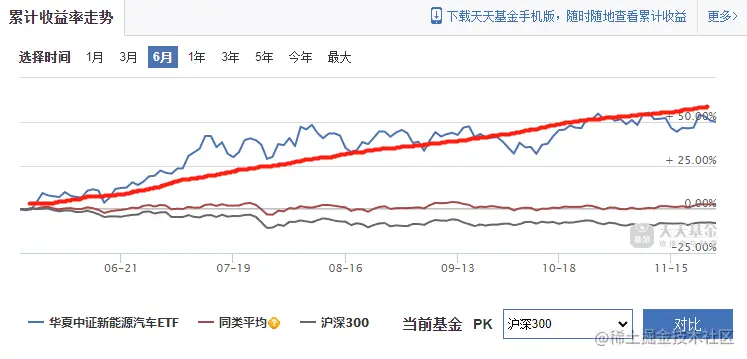The model for analyzing the fund trend here adopts linear regression , Assume that its trend is consistent with $\ce{ y = kx + b }$, y Is the corresponding rate of return , x For time .k The value is the slope . What we need to do now is to use the data of this group of funds to calculate this k value , So you can use this k Compare the value of the Fund .

#### 3 Data capture and analysis

##### 3.1 Fund data capture

Grab the data of the fund's historical rate of return

#  Grab the historical rate of return data connection of the fund
http://api.fund.eastmoney.com/pinzhong/LJSYLZS?fundCode=515030&indexcode=000300&type=y
#  Parameter description
fundCode  For the fund code to be queried
indexcode  Fund comparison benchmark data , The default is Shanghai and Shenzhen 300(000300)
type  For the period of data query ,m  A month  q 3 Months  hy 6 Months  y  A year  try 3 year  fiy 5 year  sy  This year  se  Maximum
Copy code 

stay api In the data returned by the interface ,0 Represents fund data , 1 Is the average of similar funds ,2 Shanghai and Shenzhen 300 The data of .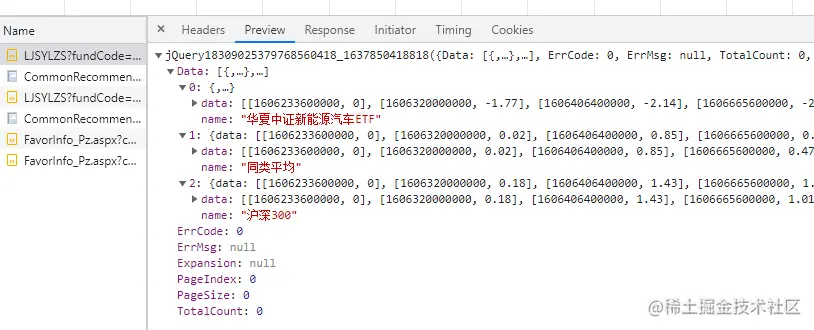The specific implementation code is shown in Figure :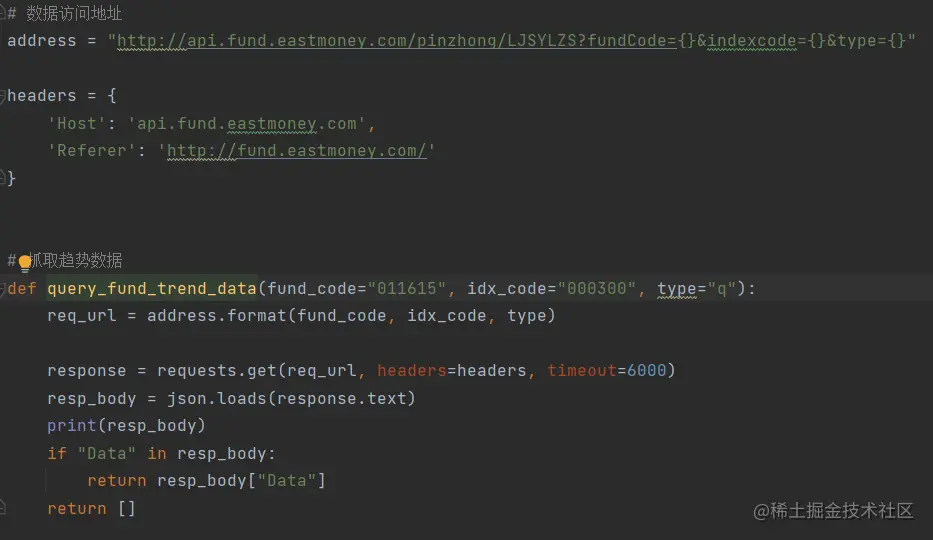##### 3.2 Data analysis

The way of data analysis is to use matplotlib and sklearn.linear_model , The first is the graphical presentation of data , The second is the linear analysis tool , Used to calculate the fund k value . About linear analysis , If you are interested, you can query the calculation details of linear analysis .

As shown in the figure below , Code for data model calculation and graphical presentation .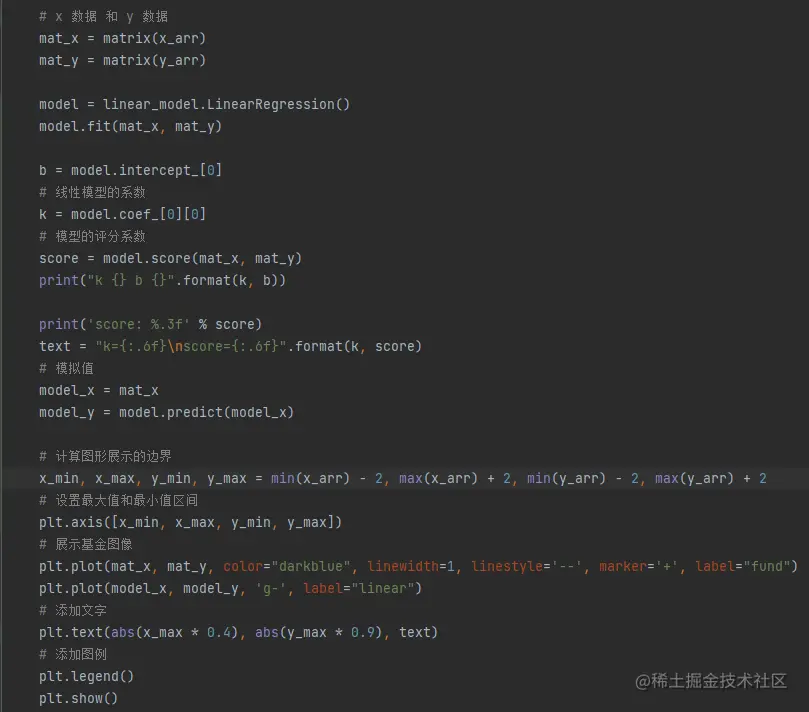New energy ETF Take the data , We got y= 0.3541x + b The trend line , The score of this linear model is 0.741. In fact, this score is already quite high , The higher the yield , The greater the fluctuation , The lower the fit with linear programming .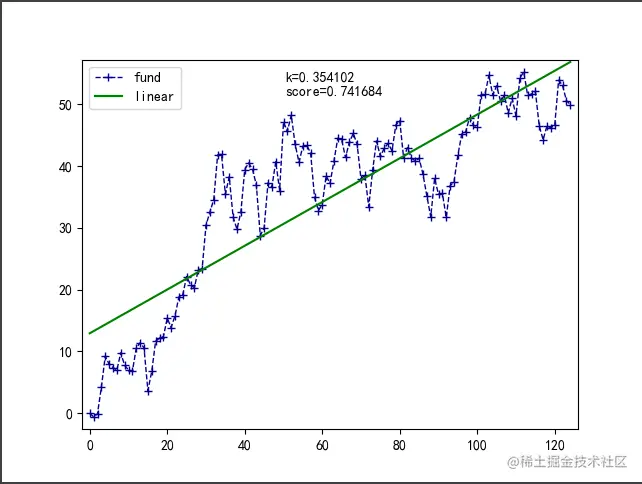But are there any exceptions , Increase profits and short-term debts with Tianhong C(008647) For example , The score is quite high , Look at the graphic display, you can know , But bond funds k It's worth more than a stock fund k The value ratio is quite low , High risk , High return , Low risk , Low return . Return is compensation for risk .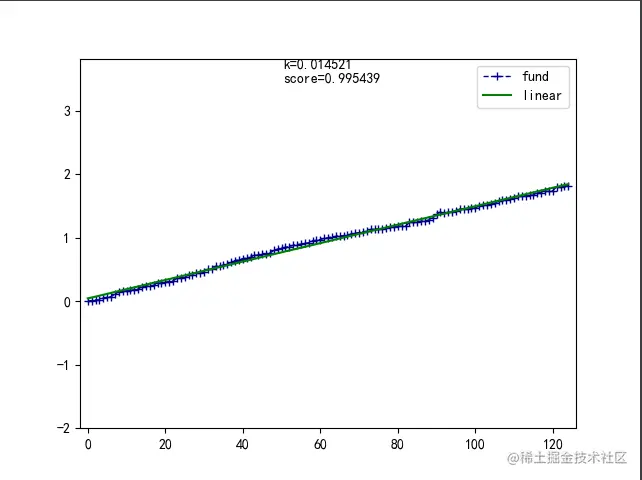#### 4 summary

In this chapter , This paper introduces the use of linear programming to analyze the trend of funds , And use the method of quantitative analysis to analyze and screen the fund . Finally, this method can be used to analyze all funds , Select funds with strong trend for investment .